# Category Archives: World Of Arduino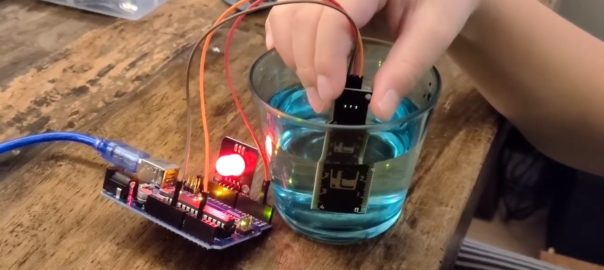# Water Level Sensor

We tried the water level sensor today and using a RGB to indicate different water level, let us show you how to do this.

## How does water level sensor work

When we use the water level sensor, we need to connect to 5V, GND and Signal to the Arduino board. It contains ten copper strips in the sensor area that are actually connected to the 5V and signal, they interlace to each others. When we immerse the sensor into water (or solution), it induces conductivity, i.e. current. The more the sensor immerse into the water, the higher conductivity will be resulted. It gives a higher signal back to the analog input in the Arduino board or what board you are using.

## Measurement and calibration

Since the water level senor will give the signal in an analog number, but not the actual water level. We need to correlate the analog number to the water level. For example, when we immerse the water sensor into 20mm of the water it will give a signal of 600. So, we wrote a function to measure the analog number for each water level we need.

```void calibration(){
int i;

digitalWrite(2,HIGH);

for (i=0;i<=4;i++){
Serial.print("put your sensor in the '");
Serial.print(i);
Serial.print("' mark");
delay(2000);
Serial.print(" ");
Serial.println(water_mark[i]);

}

Serial.println("Calibration Complete");
delay(3000);
}```

We created a function – calibration() to record the analog number for each different water levels, we defined an array water_mark[] to record them. We needed to record 4 different levels, using a for loop to read the levels one by one from analogRead(A2) as we connected the water level sensor to analog input pin – A2. We also used the Serial.print to communicate with the user so that the user knows where he has to put the water level sensor in order for the program to read the water level accurately.

```void RGB_water_sensor(int vol){
digitalWrite(12,HIGH);

if (vol < water_mark){
analogWrite(Red,255);
analogWrite(Blue,255);
analogWrite(Green,255);
}
if (vol >= water_mark && vol < water_mark){
analogWrite(Red,0);
analogWrite(Blue,0);
analogWrite(Green,0);
}
if (vol >= water_mark && vol < water_mark){
analogWrite(Red,255);
analogWrite(Blue,255);
analogWrite(Green,0);
}
if (vol >= water_mark && vol < water_mark){
analogWrite(Red,255);
analogWrite(Blue,0);
analogWrite(Green,255);
}
if (vol >= water_mark){
analogWrite(Red,0);
analogWrite(Blue,255);
analogWrite(Green,255);
}
}```

Then, we used the information from the calibration() function to define which water level we use to make the RGB light change. When we call this function – RGB_water_sensor(int vol), we need to provide the existing water level value. It will put into variable vol and compare with different water_mark[] we captured in the calibration() function. If vol (the existing water level) below the water_mark, it will be no display. If vol between water_mark and water_mark, the color is white. It will be green when between water_mark and water_mark. Then, will be blue and red colors for between 3 & 4 and over 4.

See our separated post for how RGB working.

## Conductivity of different liquid

We conducted a small experiment by comparing the measuring value between Water, Coca Cola, Perrier, Energy Drink and Vinegar, took 10 set data of each and compared the average value. Do you know which one get the best conductivity?

We thought that Lucozade has the best conductivity because it is ‘energy drink’. However, based on the experiment, the best liquid conductivity level is vinegar, and the Perrier is the worst. It is because vinegar contains acid ions but surprised us that Perrier is the worst, it should contain minerals which help conductivity. Moreover, what we observed is that when we put water in the 5mm mark of the water sensor it is the lowest conductivity out of all the liquid we used.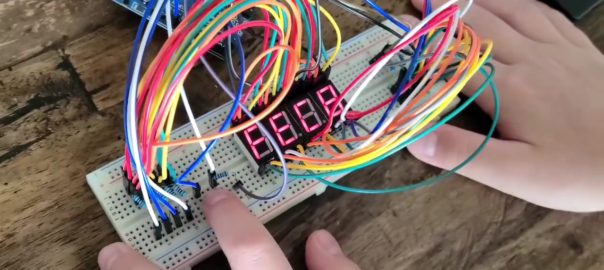# 7 Segment DEC & HEX counter

This is our second Arduino project, using 4 piece 7-segment LED display to create a DEC / HEX counter which can support both common anode and common cathode components.

## 7 Segment Display

See the photo for how we connect the 7 segment display, you need to connect a – g & DP to the corresponding pin in the Arduino board to turn each them on & off. Then, you need to connect the common to the GND or 5V+, which depends on which type of diode you are using, i.e. Common Anode or Common Cathode. The one we are suing in this project is common cathode. But, we don’t connect this to ground, we will explain later.

SO, if you want to display a number of 5, what you need to do is..

Common = GND, a = 1, b = 0, c = 1, d = 1, e = 0, f = 1, g = 0

## How to connect four displays separately?

Since we were making a 4 digits counter with four separated display, we need to control total 7 x 4 ports without the DP as well as a switch for doing inputs, it’s 29 ports. However, our Arduino only can support 13 ports. How can we control all of them?

We use the common cathode (GND) as the control, any idea? For a common cathode display, it is only working when the common connect to GND or a LOW port. What will happen if we give a HIGH (5v) to common? The answer is, it will not be working no ever what signal generates to a – g & DP port. So, we are using the common as a switch between each single 7 segment display.

You can take a try with one 7 segment display, connect the common to a port instead of GND. Then, see the different for common = high and common = low.

Since we can control 4 displays on & off, we can use 7 ports to generate signal to all four display. Then, switch on the one you want to display. We have now,

• Port 2 – 8 connect to a – g for all four displays
• Port9 – display one, one place
• Port 10 – display two, tenth place
• Port 11 – display three, hundredth place
• Port 12 – display three, thousandth place

If we want to display ‘5’ in the hundredth place, it will be

• Port 2 – 8 = 1, 0, 1, 1, 0, 1, 0
• Port 10 = 1
• Port 11 = 1
• Port 12 = 0
• Port 13 = 1

If you keep all the above and change Port 13 = 0, both thousandth and hundredth place will display 5. See?

## How can we see different numbers the same time?

Do you know ‘Persistence of vision’? When you see something, the image keep in your eye for a short period of time prior it disappear, normally 1/16 second. If there is the second image come into your eye before the last one disappear, you will see them appear ‘the same’ time, try to flickering your finger quickly and see what happen. So, if you keep displaying something within 1/16 sec, you will see all of them. Got it?

Yes, if we can display all four numbers within 1/16 sec. Your eyes and brain is being cheated to believe that all four numbers are displaying the same time. But actually, what we program is switching display one by one in a very high speed.

## Our program features

We made a 4 digits counter with the following features,

1. It support 7 segment set with common anode or common cathode, but not mix.
2. It can set how much it count, input the increment need.
3. Count in decimal from 0 – 9999 or hexadecimal #0 – #FFFF.
4. Pause the count when switch press.

Keep in mind, I have port 2 – 8 connect to a – g, port 9 for a switch and port 10 – 13 connect to common of displays.

### Individual number display

```// display a single digit with the specified digit place (0 - 3) and number (0 - 0)

void digit_display(int place, int number){
int i = 0;
const int number_count_array= {
{a,a,a,a,a,a,b},
{b,a,a,b,b,b,b},
{a,a,b,a,a,b,a},
{a,a,a,a,b,b,a},
{b,a,a,b,b,a,a},
{a,b,a,a,b,a,a},
{a,b,a,a,a,a,a},
{a,a,a,b,b,b,b},
{a,a,a,a,a,a,a},
{a,a,a,b,b,a,a},
{a,a,a,b,a,a,a},
{b,b,a,a,a,a,a},
{a,b,b,a,a,a,b},
{b,a,a,a,a,b,a},
{a,b,b,a,a,a,a},
{a,b,b,b,a,a,a},
{b,a,a,a,a,b,a},
{b,b,a,b,a,a,a},
{b,b,b,b,b,b,a}
};
digitalWrite(led_1,a);
digitalWrite(led_2,a);
digitalWrite(led_3,a);
digitalWrite(led_4,a);
digitalWrite(led_1+place,b);

for(i=0;i<=6;i++){
digitalWrite(start_pin+i,number_count_array[number][i]);
}
for(i=0;i<=6;i++){
digitalWrite(start_pin+i,b);
}
}```

We defined 0 – F including three special character h, d & ‘-‘ into an array – number_count_array[], using a & b instead of 1 & 0 because we wrote this program to support both common anode and common cathode display. If the display is common anode, will define a = 0 & b = 1. Otherwise, it will be a = 1 & b = 0 for common cathode. When the function being called, we need to provide digit place and number to be display, i.e. digit_display(0,5), will display 5 at 1st display, i.e. one place. Once the number is displayed, it need to be erased prior exit the function.

### 4 digits number display

```// display a 4 digits number and how long for the display stay, i.e delay target, 100 = 0.1s, 1 = 0.001s

void four_digit_display(unsigned int number, int delay_target){
int th; // thousandth place
int h; // hundredth place
int t; // tenth place
int o; // ones place
int d;

th = number / pow(number_system,3);
number -= th*pow(number_system,3);

h = number / pow(number_system,2);
number -= h*pow(number_system,2);

t = number / number_system;
number -= t*number_system;

o = number;

for (d=0; d<=delay_target;d++){
if (o > 0 or t > 0 or h > 0 or th > 0){
digit_display(0,o);
}
if (t > 0 or h > 0 or th > 0){
digit_display(1,t);
}
if (h > 0 or th > 0){
digit_display(2,h);
}
if (th > 0){
digit_display(3,th);
}
delay(1);
}
}```

We need to tell this function what number to be displayed and how long it stay on the screen, the target number can be 0 – 9999 for decimal system or 0 – 65535 (#0 – #FFFF) for hexadecimal system. Once it get the number, it will identify the individual digit place and it’s number. Then, it will call the digit_display to display the individual number at the digit place one by one. Using a for to achieve the target display time, i.e. the delay_target.

### Press button control

```// port - the port connect to the switch
// value - the value you want to display when running the function
// return is the uSecond for the button pressed

int press_button(int port,int value,int routine){
int hold_button = 0;

while (true){
if (routine == 9){
four_digit_display(value, 1);
hold_button ++;
delay(1);
four_digit_display(value, 1);
return hold_button;
}
}
}

if (routine != 9){
digit_display(1,16);
digit_display(3,17);
digit_display(routine,18);
hold_button ++;
delay(1);
digit_display(1,16);
digit_display(3,17);
digit_display(routine,18);
return hold_button;
}
}
}
}
}```

That’s the press button function, we need to tell the function which port of the switch to read, what number need to display or routine ‘not 9’ for special function (that’s for DEC/HEX selection, will explain later). Then, it will return the press time, so that we can identify short press or long press that we can achieve ‘select’ and ‘confirm’ with only one button. Since we need to keep the display when waiting for the press button, that’s why we need to send the existing number to this function, it will call the four_digit_display to display the number when counting the press time.

For routine ‘not 9’, it’s actually doing the same thing to count the button press time. However, it is being call when doing DEC & HEX selection, so that the display is ‘h d-‘ instead of existing number.

### Input increment

```int increment_input(){
int confirm = false;
int hold_button;
int press_target = 100;
int place_value=0;
int increment_value = 1;
int increment = 0;

while(confirm == false){

hold_button = press_button(button, increment, 9);
four_digit_display(increment,1);

if (hold_button <= press_target && hold_button != 0){
increment += increment_value;
}

if (hold_button > press_target) {
place_value++;
increment_value *= number_system;
}

if (increment >= number_system*increment_value && place_value < 4) {
increment -= number_system*increment_value;
}

if (place_value == 4){
return increment;
}
}
}```

That’s the function to input what will be the increment when counting start from 0. As we mentioned that we will get the press time from press button function, we use short press (<100ms here) to be the number adder and long press as the confirmation. Once the function start, it is waiting for the button press, short press is rotating number from 0 – 9 (DEC) or 0 – F (HEX), i.e. see number_system later. Long press will be confirmation and go for the next place until four digit input, it will return the confirm increment number.

When we call the press button function, we need to provide the existing number to keep the number being display.

```int d_h_declare(){
int change = 0;
int hold_button;
int press_target = 100;

while(true){
digit_display(1,16);
digit_display(3,17);
digit_display(change,18);
hold_button = press_button(button,0,change);

if (hold_button <= press_target && hold_button != 0){
if (change == 0) {
change = 2;
}
else{
change = 0;
}
}

if (hold_button > press_target) {
if (change == 0){
return 10;
}
if (change == 2) {
return 16;
}
}
}
}```

A function to select between decimal or hexadecimal, a ‘h d-‘ will be displayed. Short press to select between h(HEX) and d(DEC), ‘h d-‘ or ‘h-d ‘. Long press to confirm. Once confirm, it will return 10 or 16 into the number_system, it is global variable.

### Common anode and common cathode

```int Common_GND_or_Common_Anode(){
int hold_button;
int press_target = 150;

while(true){
hold_button = press_button(button,2,9);
if (hold_button <= press_target && hold_button != 0){
if (a == 1){
a = 0;
}
else{
a = 1;
}
if (b == 0){
b = 1;
}
else{
b = 0;
}
}
if (hold_button > press_target) {
break;
}
}
}```

We tried to make my program to support both common anode and common cathode display. Since we used common cathode display to build my circuity, common cathode was defined as default. When it start, it will display a ‘2’ if you connect to common cathode display or ‘000 ‘ if you connect to common anode. Use short press to switch between ‘2’ & ‘000 ‘, long press to confirm once ‘2’ is displayed. Then, the program will switch a & b (check back individual number), common anode – a = 0, b = 1, common cathode – a = 1, b = 0.

It will be appreciated if you can share with me idea for how to detect common anode and common cathode automatically.

### Let’s start

We have everything be ready but the main counting program… it is easy. Prior you start this, do the setup program in the following steps,

1. Confirm common anode or common cathode
2. Which number system you need to count – decimal or hexadecimal
3. Input the target increment value
4. Start the counting loop!

### Easier Life

Actually, it is much easier to make the circuity by using a 4-in-1 7 Segment display or even some other display module, controller like MAX7219. But, it was really fun for us to start from very basic, making a ‘time bomber’ like counter. Hope you enjoy this project.

Working on the controller like MAX7219 is something new and will be interesting too.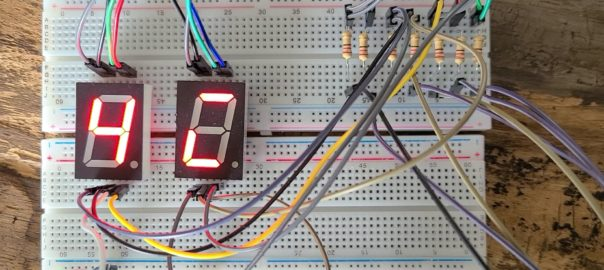# Anode vs Cathode

When you deal with diode or transistor components such as LED, 7 Segment display, RGB LED, etc., you need to understand whether the component is common anode or common cathode, it will give you completely different result.

## 7 Segment Display

Let’s us the 7 Segment display as example.

All a – g & dp are connected to a ‘common’. When the common is common anode, we need to connect this to a high voltage, sometime 5V, so that current will be generated when a – g & dp is LOW. On the other hand, if the common is common ground, we need to connect this to a low voltage, most of the time is ground, so that current will be generated when a – g & dp is HIGH.

Common anode – the segment will be lit if the signal pins a – f & dp is LOW

Common cathode – the segment will be lit if the signal pins a -f & dp is HIGH.

As you can see from the above photos, left display is common cathode and right one is common anode. When we display a number 4 to the common cathode display, it will give the opposite result from the common anode. If you display a number 4 in the common anode device, it will give the same opposite result in common cathode device.

## Switching between 1 & 0

So, when you develop the program, you need to understand what device is going to use. Otherwise, you need to do some switching between 1 & 0, just like the program below.

```int start_pin = 2;

void setup() {
// put your setup code here, to run once:
int p;
for (p=start_pin; p<start_pin+7; p++){
pinMode(p, OUTPUT);
}
}

void loop() {
// put your main code here, to run repeatedly:
int i = 0;
int j = 0;
int number = 0;
int d = 0;
int segment = 0;
const int number_array[]={{1,1,1,1,1,1,0},
{0,1,1,0,0,0,0},
{1,1,0,1,1,0,1},
{1,1,1,1,0,0,1},
{0,1,1,0,0,1,1},
{1,0,1,1,0,1,1},
{1,0,1,1,1,1,1},
{1,1,1,0,0,0,0},
{1,1,1,1,1,1,1},
{1,1,1,0,0,1,1}
};

for (number=0;number<10;number++){
for (d=0;d<100;d++){
for (segment=0;segment<8;segment++){
digitalWrite(start_pin+segment, number_array[number][segment]);
}
delay(10);
}
}

for (number=0;number<10;number++){
for (d=0;d<100;d++){
for (segment=0;segment<8;segment++){
digitalWrite(start_pin+segment, not number_array[number][segment]);
}
delay(10);
}
}
}```

This program is developed with common cathode assumed, the number_array with 1 is on, 0 is off, i.e. 4 is 0110011. When apply the same pattern to the common anode device, it will look like ‘1001100’, the monster you see in those picture.

So, we add a ‘not’ to the line we highlighted in the code, it will give the opposite value to the pin, i.e. 1 -> 0, 0 -> 1. Thus, the data output of 4 will be changed from ‘0110011’ to ‘1001100’, it will be 4 in the common anode but a monster in common cathode.

## RGB LED

7 segment is quite easy to hand. But, take a look of RGB LED. I would prefer using common cathode RGB LED, because it is easy for me just follow the color code. But for common anode one, you need to do something like 255 – the color code as shown below, have a try!

```  analogWrite (red, 255 - 232); # Color code 232, 97, 0 (E86100)
analogWrite (green, 255 - 97);
analogWrite (blue, 255 - 0);
delay(1000);

analogWrite (red, 255 - 199); # Color code 199, 128, 35 (C78023)
analogWrite (green, 255 - 128);
analogWrite (blue, 255 - 35);
delay(1000);  ```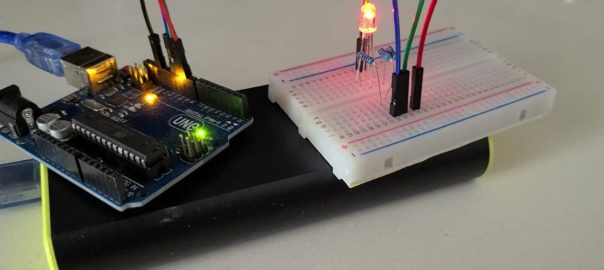# RGB LED

R – Red, G – Green, B – Blue, basic display component to display color. We call this as one pixel for all display unit, such as monitor, TV and smart phone. It contains three inputs for red, green and blue, as well as a common ground(1). When we apply ‘analog’ voltage(2) to each corresponding pin, it will display the corresponding scale of the color. For Arduino, it can provide 0-255 scale of ‘analog’ voltage to the pin, i.e. we can get 256 different color scales of red, green or blue. If we put them together, it will give 256x256x256 = 16,777,216 colors!

(1) Do you know common anode and common cathode? see our separated post.

```void loop() {

analogWrite (red, 255);
analogWrite (green, 0);
analogWrite (blue, 0);
delay(1000);

analogWrite (red, 0);
analogWrite (green, 255);
analogWrite (blue, 0);
delay(1000);

analogWrite (red, 0);
analogWrite (green, 0);
analogWrite (blue, 255);
delay(1000);

analogWrite (red, 0);
analogWrite (green, 0);
analogWrite (blue, 0);
delay(1000);

analogWrite (red, 255);
analogWrite (green, 255);
analogWrite (blue, 255);
delay(1000);

analogWrite (red, 232);
analogWrite (green,97);
analogWrite (blue, 0);
delay(1000);

analogWrite (red, 255);
analogWrite (green,0);
analogWrite (blue, 255);
delay(1000);
}```

Since we need to provide ‘analog’ voltage to the RGB LED, we must be using port with ‘analog’ output support that you can see ‘~’ next to the port number, so that you can write 0 – 255 to the port to control color scale to generate different color and achieve different colors combination. Refer to above table for examples of color combination (we call this color code) or the link to a color code web site. All the color is the combination of RGB in term of hexadecimal number, e.g. #FF is 255, color code of red is #FF0000, color code of Orange is #E86100 where #E8 = 232, #61 = 97, #00 = 0.

## What is pixel?

HD? 2K? 4K and 8K? When we talk about the monitor or TV, it’s quality is always related to the screen resolution, the higher resolution, more pixels, higher density, the better of the screen quality. For a 8K TV, it contains more than 33million tiny and very high quality RGB LED!!! That’s not easy to produce with good yield, that’s why it is very expensive!!!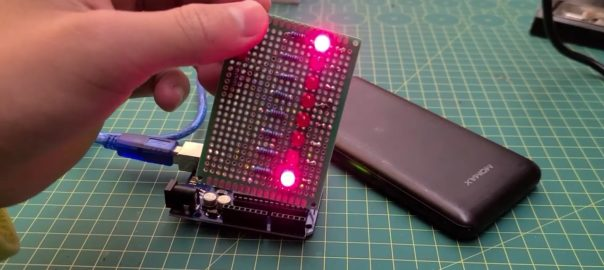# Our First PCBA

After we learnt some basic idea about the Arduino UNO board and basic LED circuitry, we started to build our first project – LED array. But, keep in mind, always put loading (i.e. resistor) for LED. Otherwise, you will burn it or even damaged the board.

We built LED array circuitry with the ‘bread’ board, so that we can easily adjust and debug the circuitry prior fit them onto a PCB.

We also learnt how to connect the switch to the input pin with pull-up and pull down resistor concept. So that the input pin will not be floating with uncertain voltage which will induce unstable the result. We used pull-down resistor because we want to trigger the LED pattern when the switch is being pressed.

```void turn_on() {

int Light_effect = 1 ;

if (button == HIGH ){
Light_effect = Light_effect + 1;
if (Light_effect == 4) {
Light_effect = 1 ;
}
}
if (Light_effect == 1) {
Light_1();
}
if (Light_effect == 2) {
Light_2();
}
if (Light_effect == 3) {
Light_3();
}
}  ```

We defined 3 lighting effects by on/off different port of LED in preset sequence. When the switch connect to port 10 is pressed, it will change the light effect value and call the function for the corresponding light effect. Once the light effect 3 is done, it will switch back to the first one. Below is the first light effect, try to create your own and simplify the coding.

```void Light_1() {

digitalWrite (9,HIGH) ;
delay (100) ;
digitalWrite (8,HIGH) ;
delay (100) ;
digitalWrite (7,HIGH) ;
delay (100) ;
digitalWrite (6,HIGH) ;
delay (100) ;
digitalWrite (5,HIGH) ;
delay (100) ;
digitalWrite (4,HIGH) ;
delay (100) ;
digitalWrite (3,HIGH) ;
delay (100) ;
digitalWrite (2,HIGH) ;
delay (100) ;
digitalWrite (9,LOW) ;
delay (100) ;
digitalWrite (8,LOW) ;
delay (100) ;
digitalWrite (7,LOW) ;
delay (100) ;
digitalWrite (6,LOW) ;
delay (100) ;
digitalWrite (5,LOW) ;
delay (100) ;
digitalWrite (4,LOW) ;
delay (100) ;
digitalWrite (3,LOW) ;
delay (100) ;
digitalWrite (2,LOW) ;
delay (100) ;
}```

Once we confirmed the circuitry is good and the program is running well, we soldered all the components to a double side PCB. We made our first PCBA without being scalded by the soldering iron!!!